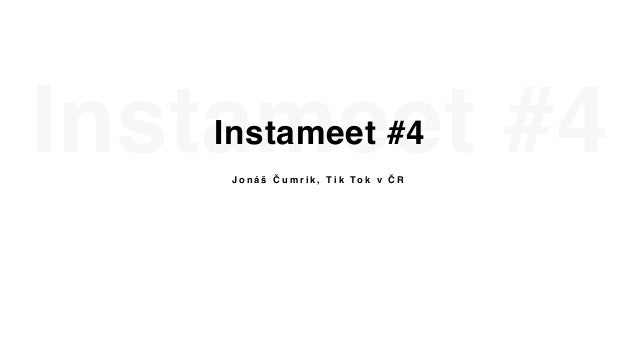Successfully reported this slideshow.Upcoming SlideShare
×

# Johny Machette / Jonas Cumrik - Instameet 2020

184 views

Published on

Základy využívání sítě Tiktok a case study o spuštění profilu Universal Music v Česku představil Youtuber v důchodu Jonáš Čumřík alias Johny Machette.

Instameet 2020, Máj 4. patro
www.instameet.cz ⠀⠀

Published in: Marketing
• Full Name
Comment goes here.

Are you sure you want to Yes No• Be the first to comment

• Be the first to like this

### Johny Machette / Jonas Cumrik - Instameet 2020

1. 1. Instameet #4Instameet #4 J o n á š Č u m r i k , T i k To k v Č R
2. 2. VYSLOVUJE SE TO:VYSLOVUJE SE TO: [ Ty k To k ] Nemáte zač, bůmři :)))
3. 3. Jsou tam jen děti?Jsou tam jen děti? V í c e n e ž 5 0 % u ž i v a t e l ů v Č R j e s t a r š í 1 8 l e t
4. 4. DALŠÍ ČÍSLA….DALŠÍ ČÍSLA 3 - p ř e s n ě t o l i k k o n t r o l č e k á v á š c o n t e n t n e ž s e d o s t a n e n a F o r Yo u p a g e
5. 5. TVŮRCI NA TTTVŮRCI NA TT T O P 5 v Č R
6. 6. INFLUENCER SNŮINFLUENCER SNŮ K d y ž s e z e z a m ě s t n a n c e s t a n e i n f l u e n c e r < 3
7. 7. FIRMY NA TTFIRMY NA TT p a r d o n , p o k u d j s e m n a v á s z a p o m n ě l ! - H o b ž o v i s t r á ž n i c k é b r a m b ů r k y - E l y M a n a g e m e n t - C a n o n
8. 8. FIRMY NA TTFIRMY NA TT - @ A m a z o n p r i m e v i d e o - @ Vo g u e p a r i s - @ Ta s t e m a d e - @ S e p h o r a
9. 9. BOOMER JONÁŠBOOMER JONÁŠ @ d z o n y m a c e t a Z a v š e c h n o m o h l a k a r a n t é n a !
10. 10. BOOMER JONÁŠBOOMER JONÁŠ D ATA 5 0 k v i e w s - Ž u f á n e k 1 5 0 k v i e w s - C o c a - C o l a
11. 11. UNIVERSAL MUSICUNIVERSAL MUSIC @ u m u s i c c z Červen 2020
12. 12. JAK ZAČÍT?JAK ZAČÍT? C o n t e n t
13. 13. “COŽE”“COŽE” C o n t e n t z a d a r m o !
14. 14. UNIVERSAL MUSICUNIVERSAL MUSIC @ u m u s i c c z 3 2 v i d e i 8 2 6 k v i e w s 3 2 5 0 v i d e í v y t v o ř i l i n a n á š s o u n d u ž i v a t e l é t t z Č R / S K 5 v i d e í o d I n f l u e n c e r ů
15. 15. ŠUP PRYČ!ŠUP PRYČ! Ž e n e m e l i d i n a j i n é p l a t f o r m y 1 0 k + v i d e í n a s o u n d ( 2 m i l v i e w s j e n v T T ) T O P 5 0 n a S p o t i f y T O P 1 0 n a Y T B E ( t r e n d y )   2 , 5 m i l m o n e t i z o v a n ý c h v i e w s ( Y T B E + S p o t i f y ) - n e z á v i s l ý u m ě l e c - z e 3 k n a 7 0 k p o s l u c h a č ů m ě s í č n ě ( S p o t i f y )
16. 16. @ J o h n y M a c h e t t e J o n á š Č u m r i k